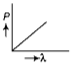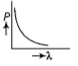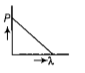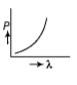The maximum kinetic energy of photoelectron emitted from the surface of work function f due to incidence of light of frequency n is E. If the frequency of incident light is doubled, then maximum kinetic of emitted photon will be

1.  2E

2.  2E - f

3.  2E + f

4.  2E + 2f

Concept Questions :-

Einstein photo-electric equation
NEET 2021 - Achiever Batch - Aryan Raj Singh

Difficulty Level:

The de-Broglie associated with an electron accelerated through a voltage of 900 V is:

1.

2.

3.

4.

Concept Questions :-

De-broglie wavelength
NEET 2021 - Achiever Batch - Aryan Raj Singh

Difficulty Level:

The de-Brogile wavelength of a neutron in thermal equilibrium with heavy water at a temperature T (Kelvin) and mass m, is

(a)$\frac{h}{\sqrt{mkT}}$

(b)$\frac{h}{\sqrt{3mkT}}$

(c)

(d)$\frac{2h}{\sqrt{mkT}}$

Concept Questions :-

De-broglie wavelength
NEET 2021 - Achiever Batch - Aryan Raj Singh

Difficulty Level:

Electrons of mass m with de-Broglie wavelength $\lambda$ fall on the target in an X-ray tube. The cut off wavelength $\left({\lambda }_{0}\right)$ of the emitted X-ray is -

(a) ${\lambda }_{0}=\frac{2mc{\lambda }^{2}}{h}$          (b) ${\lambda }_{0}=\frac{2h}{mc}$

(c) ${\lambda }_{0}=\frac{2{m}^{2}{c}^{2}{\lambda }^{3}}{{h}^{2}}$       (d) ${\lambda }_{0}=\lambda$

Concept Questions :-

Davisson and Germer experiment
NEET 2021 - Achiever Batch - Aryan Raj Singh

Difficulty Level:

Photons with energy 5 eV are incident on a cathode C in a photoelectric cell. The maximum energy of emitted photoelectrons is 2 eV. When photons of energy 6 eV are incident on C, no photoelectric will reach the anode A, if the stopping potential of A relative to C is

1. +3 V             2. +4 V

3. - 1V              4. -3 V

Concept Questions :-

Einstein photo-electric equation
NEET 2021 - Achiever Batch - Aryan Raj Singh

Difficulty Level:

When a metallic surface is illuminated with radiation of wavelength $\lambda$, the stopping potential is V. If the same surface is illuminated with radiation of wavelength 2$\lambda$, the stopping potential is $\frac{V}{4}$ .The threshold wavelength for metallic surface is:

(a) 5$\lambda$                (b)$\frac{5}{2}$$\lambda$

(c) 3$\lambda$                (d) 4$\lambda$

Concept Questions :-

Photoelectric effect experiment
NEET 2021 - Achiever Batch - Aryan Raj Singh

Difficulty Level:

An electron of mass m and a photon have same energy E. Find the ratio of de-Broglie wavelengths associated with them -(c being the velocity of light)

Concept Questions :-

De-broglie wavelength
NEET 2021 - Achiever Batch - Aryan Raj Singh

Difficulty Level:

A radiation of energy 'E' falls normally on a perfectly reflecting surface. The momentum transferred to the surface is (c=velocity of light)

1. E/c

2. 2E/c

3. 2E/c2

4. E/c2

Concept Questions :-

Particle nature of light
NEET 2021 - Achiever Batch - Aryan Raj Singh

Difficulty Level:

A certain metallic surface is illuminated with monochromatic light of wavelength λ. The stopping potential for photoelectric current for this light is 3Vo. If the same surface is illuminated with light of wavelength 2λ. the stopping potential is Vo. The threshold wavelength for this surface for the photoelectric effect is:

1. 6λ

2. 4λ

3. λ/4

4. λ/6

Concept Questions :-

Einstein photo-electric equation
NEET 2021 - Achiever Batch - Aryan Raj Singh

Difficulty Level:

Which of the following figures represent the variation of particle

momentum and the associated de-Broglie wavelength?

1.2.3.4.Concept Questions :-

De-broglie wavelength